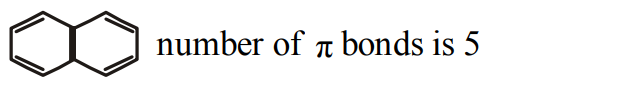## Chemical Bonding and Molecular Structure Questions and Answers Part-1

1. Chemical bond implies
a) repulsion
b) attraction
c) attraction and repulsion balanced at a particular distance
d) attraction and repulsion

Explanation: At bond distance the attractive forces overweigh the repulsive forces.

2. The bonding is electrovalent in
a) NaCl
b) $Br_{2}$
c) $PF_{5}$
d) $XeF_{4}$

Explanation: In NaCl the bonding is ionic (electrovalent)

3. Bonding in ferric chloride is
a) covalent
b) ionic
c) co-ordinate
d) None of the above

Explanation: The given compound is made up of two elements that is Ferric which is nothing but iron and thus a metal, whereas another is Chlorine which is non-metal. As we know that Ionic bonding happens with metals and non-metals, so the bond between ferric chloride is ionic.

4. Which of the following species has lowest ionization potential?
a) O
b) $O_{2}$
c) $O_2^+$
d) $O_2^-$

Explanation: $\pi*2p_x^2 \pi*2p_y^1$    antibonding molecular orbitals contain three electrons in $O_2^-$. Their energy being more and ionisation potential less.

5. Polarization power of a cation increases when
a) charge on the cation increases
b) size of the cation increases
c) charge on the cation decreases
d) has no relation to its size or charge

Explanation: Polarizing power $\phi$ = charge/ radius. Thus charge on the cation increases

6. The correct order of decreasing polarisability of following ions is
a) $CI^{-}$,$Br^{-}$,$I^{-}$,$F^{-}$
b) $F^{-}$,$I^{-}$,$Br^{-}$,$CI^{-}$
c) $F^{-}$,$CI^{-}$,$Br^{-}$,$I^{-}$
d) $I^{-}$,$Br^{-}$,$CI^{-}$,$F^{-}$

Explanation: The larger the size of anion the more is its polarizability

7. Which of the following statements regarding covalent bond is not true ?
a) The electrons are shared between atoms
b) The bond is non - directional
c) The strength of the bond depends upon the extent of overlapping
d) The bond formed may or may not be polar

Explanation: Covalent bonds are directional in nature

8. Among the following, electron deficient compound is
a) $CCI_{4}$
b) $PCI_{5}$
c) $BeCI_{2}$
d) $BCI_{3}$

Explanation: $BCI_{3}$ is lewis acid and electron deficient. The octet around B is not complete

9. Consider the following statements:
(i) A sigma $\left(\sigma\right)$ bond is formed when two s - orbitals overlap
(ii) A pi $\left(\pi\right)$ bond is formed when two p- orbitals axially overlap
(iii) A $\sigma$ -bond is weaker than $\pi-$bond.
Which of the above statements is/are correct ?
a) i and ii
b) ii and iii
c) i alone
d) ii alone

10. Number of $\pi$ bonds in naphthalene are